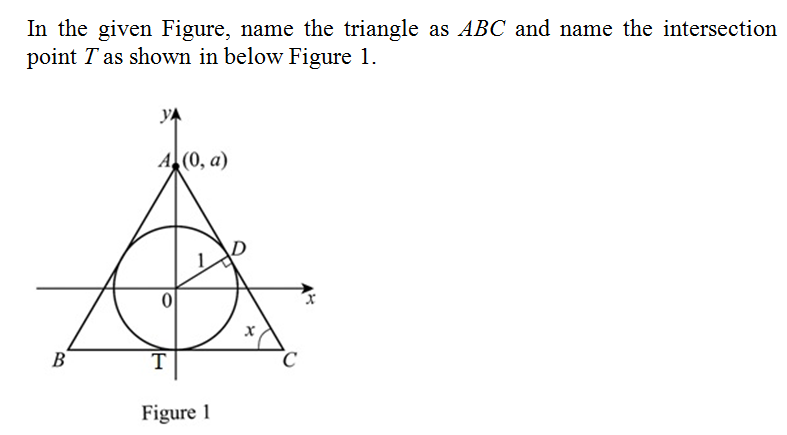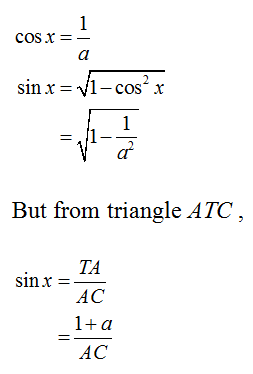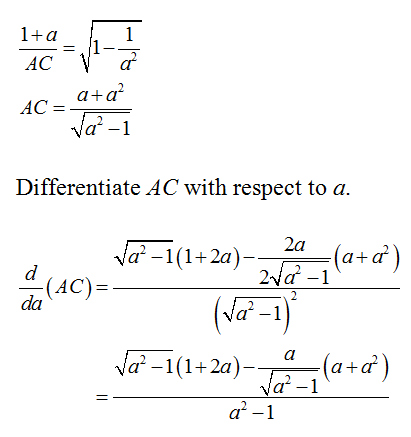Question
3 views

An isoceles triangle is circumscribed about the unit circle so that the equal sides meet at a point (0,a) on the y-axis. find the value of a that minimizes the lengths of the equal sides. (you may be surprised that the result does not give an equilateral triangle)

- I have named all sides of the triangle to make segments. A is the 3rd quadrant, B is 4th, C is (0,a), D is the intersection between A and C, E is between C and B and last F on the y-axis between A and B. I have also gotten as far to solving for CE by using Pythagorean theorem. I don't quite understand what I should do next.

check_circle

Step 1Step 2

From figure 1, it is observed that,Step 3

Compare the above two values and deduce that,...

### Want to see the full answer?

See Solution

#### Want to see this answer and more?

Solutions are written by subject experts who are available 24/7. Questions are typically answered within 1 hour.*

See Solution
*Response times may vary by subject and question.
Tagged in